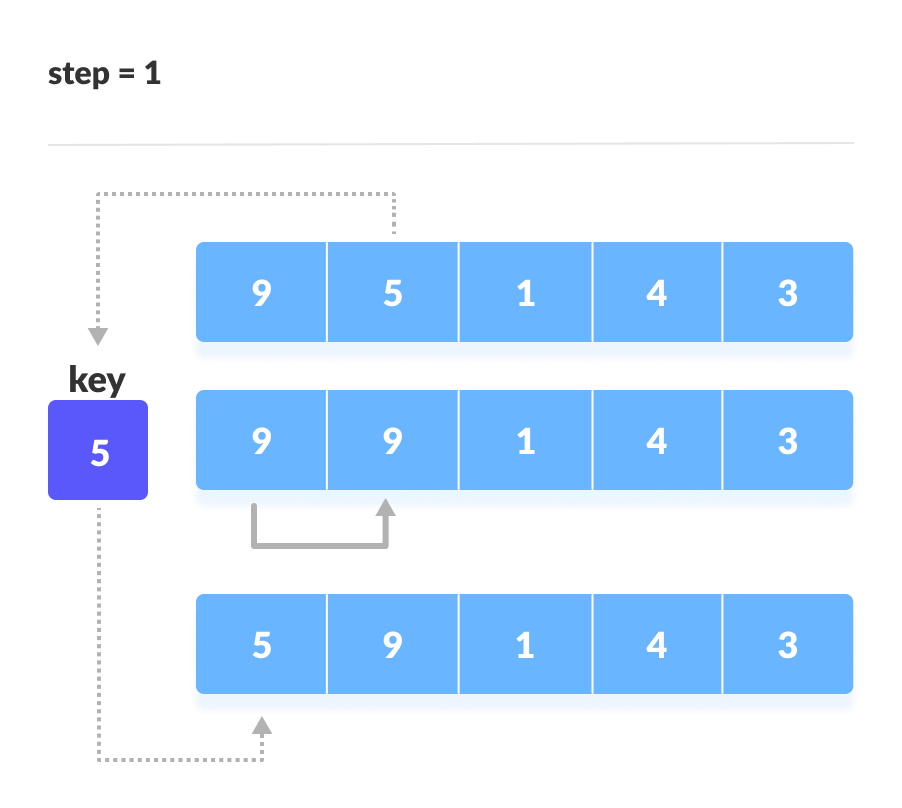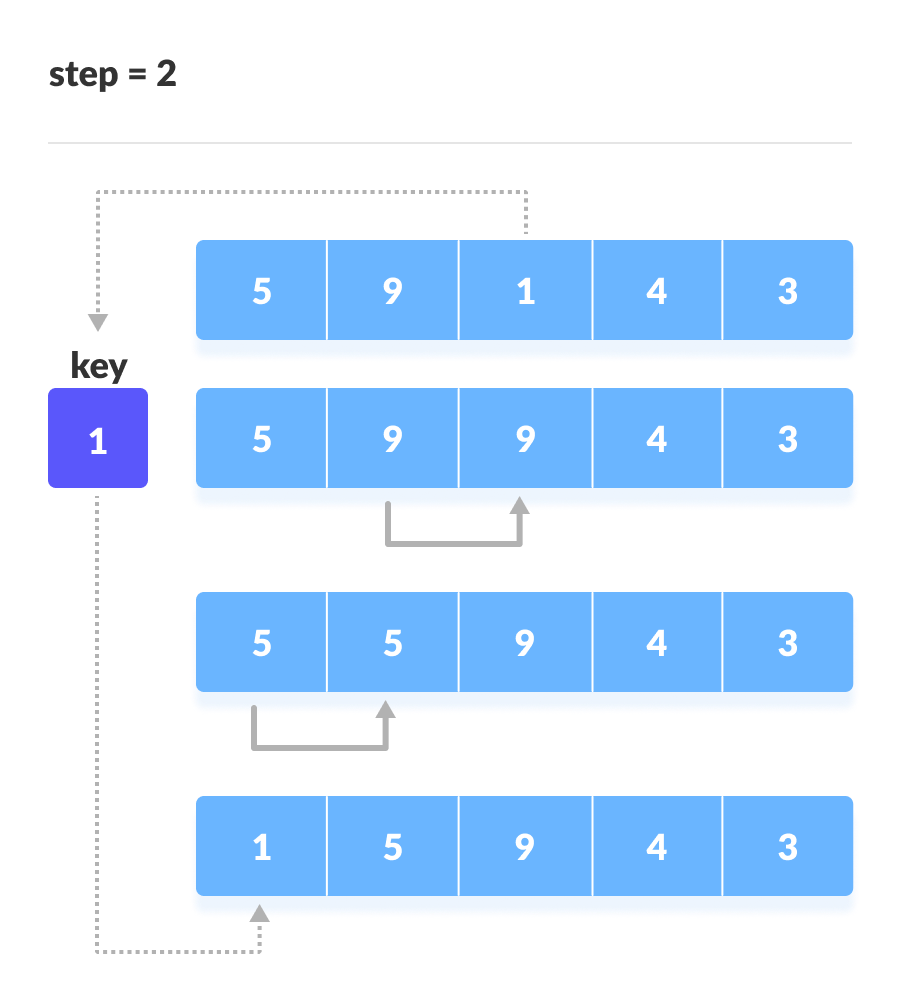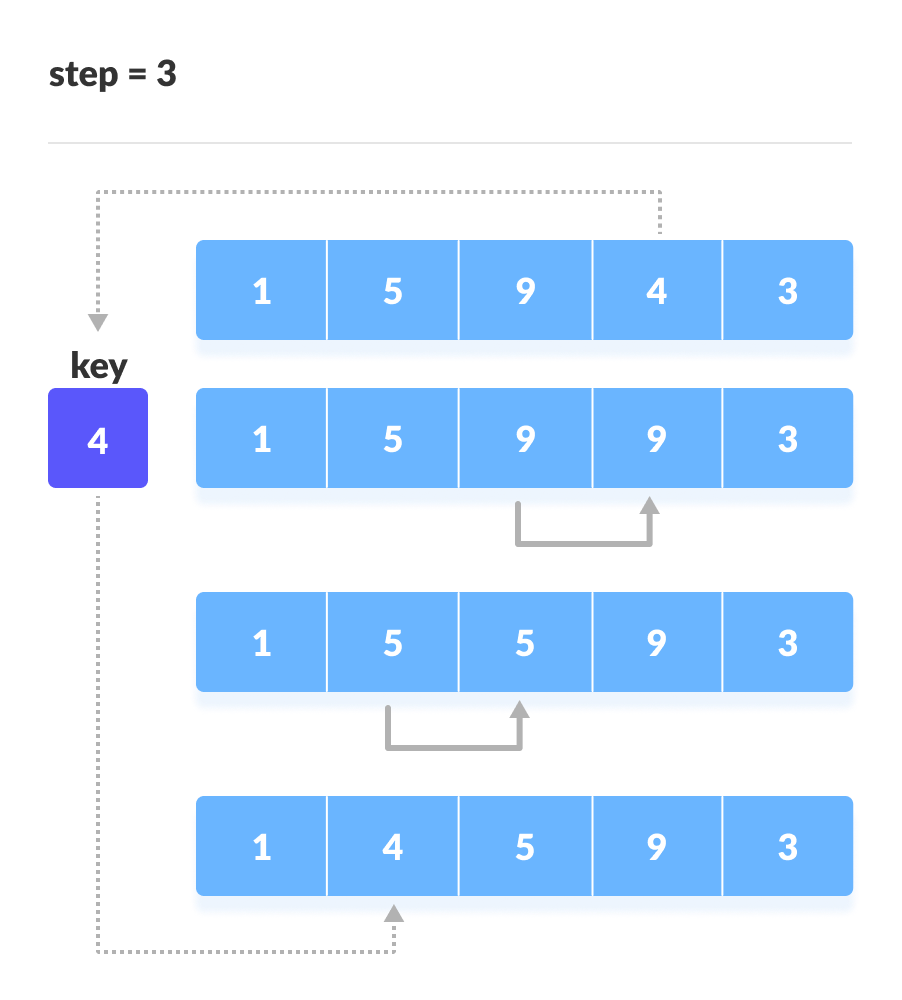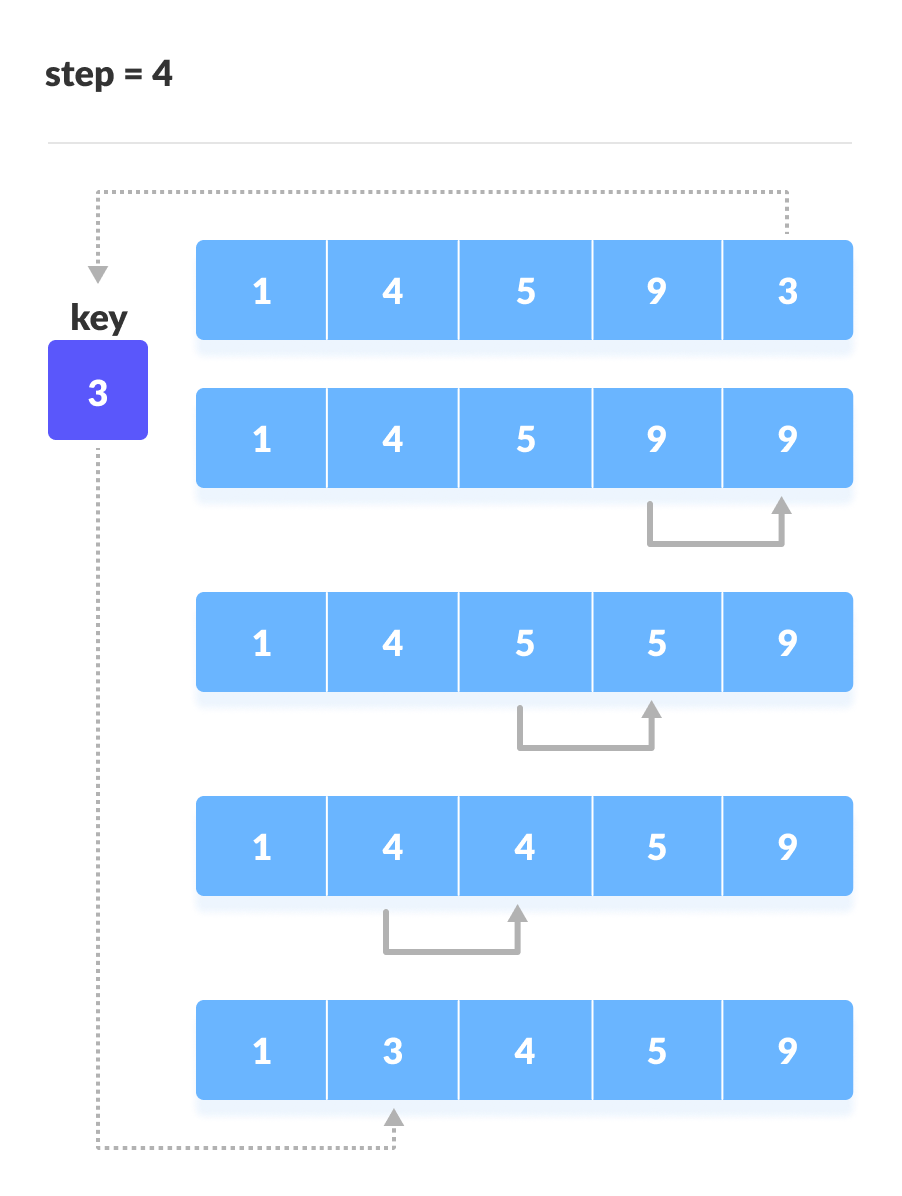Insertion Sort Algorithm

In this tutorial, you will learn how insertion sort works. Also, you will find working examples of insertion sort in C, C++, Java and Python.

Insertion sort works in the similar way as we sort cards in our hand in a card game.

We assume that the first card is already sorted then, we select an unsorted card. If the unsorted card is greater than the card in hand, it is placed on the right otherwise, to the left. In the same way, other unsorted cards are taken and put at their right place.

A similar approach is used by insertion sort.

Insertion sort is a sorting algorithm that places an unsorted element at its suitable place in each iteration.

How Insertion Sort Works?

Suppose we need to sort the following array.1. The first element in the array is assumed to be sorted. Take the second element and store it separately in key.

Compare key with the first element. If the first element is greater than key, then key is placed in front of the first elemet.2. Now, the first two elements are sorted.

Take the third element and compare it with the elements on the left of it. Placed it just behind the element smaller than it. If there is no element smaller than it, then place it at the begining of the array.3. In a similar way, place every unsorted element at its correct position.Insertion Sort Algorithm

insertionSort(array)
mark first element as sorted
for each unsorted element X
'extract' the element X
for j <- lastSortedIndex down to 0
if current element j > X
move sorted element to the right by 1
break loop and insert X here
end insertionSort

Python, Java and C/C++ Examples

# Insertion sort in Python

def insertionSort(array):

for step in range(1, len(array)):
key = array[step]
j = step - 1
while j >= 0 and key < array[j]:

# For descending order, change key<array[j] to key>array[j].

array[j + 1] = array[j]
j = j - 1
array[j + 1] = key

data = [9, 5, 1, 4, 3]
insertionSort(data)
print('Sorted Array in Ascending Order:')
print(data)
// Insertion sort in Java

import java.util.Arrays;

class InsertionSort {
void insertionSort(int array[]) {
int size = array.length;
for (int step = 1; step < size; step++) {
int key = array[step];
int j = step - 1;
while (j >= 0 && key < array[j]) {
// For descending order, change key<array[j] to key>array[j].
array[j + 1] = array[j];
--j;
}
array[j + 1] = key;
}
}

public static void main(String args[]) {
int[] data = { 9, 5, 1, 4, 3 };
InsertionSort is = new InsertionSort();
is.insertionSort(data);
System.out.println("Sorted Array in Ascending Order: ");
System.out.println(Arrays.toString(data));
}
}
// Insertion sort in C

#include <stdio.h>
void printArray(int array[], int size)
{
for (int i = 0; i < size; i++)
{
printf("%d ", array[i]);
}
printf("\n");
}
void insertionSort(int array[], int size)
{
for (int step = 1; step < size; step++)
{
int key = array[step];
int j = step - 1;
while (key < array[j] && j >= 0)
{
// For descending order, change key<array[j] to key>array[j].
array[j + 1] = array[j];
--j;
}
array[j + 1] = key;
}
}
int main()
{
int data[] = {9, 5, 1, 4, 3};
int size = sizeof(data) / sizeof(data);
insertionSort(data, size);
printf("Sorted array in ascending order:\n");
printArray(data, size);
}
// Insertion sort in C++

#include <iostream>
using namespace std;

void printArray(int array[], int size)
{
for (int i = 0; i < size; i++)
{
cout << array[i] << " ";
}
cout << endl;
}
void insertionSort(int array[], int size)
{
for (int step = 1; step < size; step++)
{
int key = array[step];
int j = step - 1;
while (key < array[j] && j >= 0)
{
// For descending order, change key<array[j] to key>array[j].
array[j + 1] = array[j];
--j;
}
array[j + 1] = key;
}
}
int main()
{
int data[] = {9, 5, 1, 4, 3};
int size = sizeof(data) / sizeof(data);
insertionSort(data, size);
cout << "Sorted array in ascending order:\n";
printArray(data, size);
}

Complexity

Time Complexities

• Worst Case Complexity: O(n2)

Suppose, an array is in ascending order, and you want to sort it in descending order. In this case, worse case complexity occers.

Each element has to be compared with each of the other elements so, for every nth element, (n-1) number of comparisons are made.

Thus, the total number of comparisons = n*(n-1) ~ n2

• Best Case Complexity: O(n)

When the array is already sorted, the outer loop runs for n number of times whereas the inner loop does not run at all. So, there is only n number of comparison. Thus, complexity is linear.

• Average Case Complexity: O(n2)

It occurs when the elements of a array are in jumbled order (neither ascending nor descending).

Space Complexity

Space complexity is O(1) because an extra variable key is used.

Insertion Sort Applications

The insertion sort is used when:

• the array is has a small number of elements
• there are only a few elements left to be sorted